https://doi.org/10.5194/bg-15-2819-2018
https://doi.org/10.5194/bg-15-2819-2018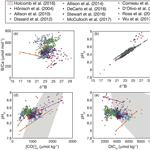# Reviews and syntheses: Revisiting the boron systematics of aragonite and their application to coral calcification

Thomas M. DeCarlo, Michael Holcomb, and Malcolm T. McCulloch
Abstract

The isotopic and elemental systematics of boron in aragonitic coral skeletons have recently been developed as a proxy for the carbonate chemistry of the coral extracellular calcifying fluid. With knowledge of the boron isotopic fractionation in seawater and the B∕Ca partition coefficient (KD) between aragonite and seawater, measurements of coral skeleton δ11B and B∕Ca can potentially constrain the full carbonate system. Two sets of abiogenic aragonite precipitation experiments designed to quantify KD have recently made possible the application of this proxy system. However, while different KD formulations have been proposed, there has not yet been a comprehensive analysis that considers both experimental datasets and explores the implications for interpreting coral skeletons. Here, we evaluate four potential KD formulations: three previously presented in the literature and one newly developed. We assess how well each formulation reconstructs the known fluid carbonate chemistry from the abiogenic experiments, and we evaluate the implications for deriving the carbonate chemistry of coral calcifying fluid. Three of the KD formulations performed similarly when applied to abiogenic aragonites precipitated from seawater and to coral skeletons. Critically, we find that some uncertainty remains in understanding the mechanism of boron elemental partitioning between aragonite and seawater, and addressing this question should be a target of additional abiogenic precipitation experiments. Despite this, boron systematics can already be applied to quantify the coral calcifying fluid carbonate system, although uncertainties associated with the proxy system should be carefully considered for each application. Finally, we present a user-friendly computer code that calculates coral calcifying fluid carbonate chemistry, including propagation of uncertainties, given inputs of boron systematics measured in coral skeleton.

Share
Dates
1 Introduction

Quantifying the carbonate chemistry of the fluid from which corals accrete their skeletons is essential for understanding the mechanisms of skeletal growth and the sensitivity of skeletal composition to environmental variability. It is generally thought that corals precipitate aragonite (CaCO3) crystals within an extracellular fluid-filled space between the living polyp and the skeleton (Barnes1970). Evidence from skeletal geochemistry and fluorescent dye experiments suggests that while seawater is the initial source of the calcifying fluid , the carbonate chemistry of the calcifying fluid is subject to substantial modifications (i.e., pH and dissolved inorganic carbon, or DIC) that enhance the rapid nucleation and growth of aragonite crystals . Because the isolation and small size of the calcifying fluid makes it difficult to sample directly, a variety of techniques have been employed to characterize its composition. These include microelectrodes inserted into tissue incisions or through the mouth , pH-sensitive dyes (; ; Comeau et al., 2017b), Raman spectroscopy , and a variety of skeletal-based geochemical proxies (; ; ; ; ; ; ). Although microelectrodes and pH-sensitive dyes are arguably the most direct methods, their utilities are limited by difficulties of applying them to corals living in their natural environment or developing seasonally resolved time series. Geochemical proxies, although indirect, can be readily applied to the skeletons of corals living in both laboratory and natural environments, and to skeletons accreted years or even centuries ago.

In recent years, boron systematics (including δ11B and B∕Ca) have become one of the most commonly applied proxies for the carbonate chemistry of coral calcifying fluid (; ; ; ; ; ; Comeau et al., 2017a; ; ; ; ; ). The sensitivity of boron isotopes to seawater pH arises from the borate versus boric acid speciation being pH dependent and the isotopic fractionation between these species being constant . Since the δ11B composition of aragonite precipitating from seawater reflects that of the borate species , the δ11B composition of the skeletal carbonate records the pH of the calcifying fluid. Furthermore, the B∕Ca ratio depends inversely on the concentration of carbonate ion ($\left[{\mathrm{CO}}_{\mathrm{3}}^{\mathrm{2}-}\right]$) if borate substitutes for carbonate ion in the aragonite lattice .

Use of combined boron isotopic (δ11B) and elemental (B∕Ca) systematics has several advantages relative to other geochemical proxies. For example, while stable carbon and oxygen isotopes are sensitive to carbonate chemistry, they are complicated by kinetic effects, strong sensitivities to the photosynthetic activity of coral symbionts, and variable compositions in seawater, which together have precluded their utility as acceptable carbonate system proxies . The U∕Ca ratio of aragonite is also sensitive to $\left[{\mathrm{CO}}_{\mathrm{3}}^{\mathrm{2}-}\right]$, but the amount of U in coral skeleton relative to its concentration in seawater suggests that [U]cf is depleted substantially, complicating its utility as a direct $\left[{\mathrm{CO}}_{\mathrm{3}}^{\mathrm{2}-}\right]$cf proxy . Conversely, the B∕Ca and δ11B compositions of seawater are homogeneous and likely not modified substantially by photosynthetic activity . Further, incorporation into the skeleton is less important for B∕Ca than U∕Ca because the partition coefficient between B and $\left[{\mathrm{CO}}_{\mathrm{3}}^{\mathrm{2}-}\right]$ is at least 2 orders of magnitude smaller than that of U ${\mathrm{CO}}_{\mathrm{3}}^{\mathrm{2}-}$ , meaning that [B]cf is depleted much less than [U]cf as skeletal aragonite precipitates. While a low partition coefficient causes Rayleigh fractionation for elements in a closed system (e.g., coral [Mg] [Ca]cf) , $\left[{\mathrm{CO}}_{\mathrm{3}}^{\mathrm{2}-}\right]$cf is elevated relative to seawater and is modified by CO2 diffusion and pH up-regulation (i.e., it is not in a closed system) , meaning that [B] $\left[{\mathrm{CO}}_{\mathrm{3}}^{\mathrm{2}-}{\right]}_{\mathrm{cf}}$ is likely not changed substantially due to skeletal aragonite precipitation. Therefore, boron-based proxies are thought to be largely dependent on carbonate chemistry alone . Finally, the combination of two carbonate system proxies (pH and $\left[{\mathrm{CO}}_{\mathrm{3}}^{\mathrm{2}-}\right]$) derived from boron systematics allows for computation of the full carbonate system .

Abiogenic laboratory experiments provide the underlying quantitative foundation necessary to apply these proxies to aragonitic coral skeletons. determined the fractionation factor (αB3−B4) between boric acid and borate in seawater, which allows δ11B of carbonates to be used as a pH proxy when combined with knowledge of pKB (Dickson1990) and seawater δ11B . Although there is potential for B isotopic fractionation between aragonite and seawater , the veracity of the δ11B proxy has been largely confirmed by comparison with direct in situ measurements using either pH microelectrodes or confocal microscopy of pH-sensitive dyes in the calcifying fluid . Additionally, results from two sets of abiogenic precipitation experiments can be used to constrain the partitioning of B∕Ca between fluid and aragonite . Thus, while all the information theoretically required to constrain the full seawater carbonate system from boron systematics is now available, a variety of different approaches have been presented, especially regarding the interpretation of B∕Ca partitioning . Here, we assess the abiogenic partitioning data , and the subsequent fitting of those data . We consider which mechanisms of B incorporation and sensitivities of B∕Ca partitioning are plausible, and the implications for interpreting coral skeletons. Our focus is on the combined application of δ11B and B∕Ca because it is only when the two are used in tandem that it is possible to calculate the full calcifying fluid carbonate system. Finally, we present a user-friendly computer code to calculate coral calcifying fluid carbonate chemistry from measurements of δ11B and B∕Ca. The code also propagates known uncertainties for deriving calcifying fluid $\left[{\mathrm{CO}}_{\mathrm{3}}^{\mathrm{2}-}\right]$cf and DICcf, and allows for evaluating the effects of using different constants and partition coefficient formulations.

2 Partitioning of B∕Ca between aragonite and seawater

The main discrepancy among various applications of boron systematics to coral skeletons relates to the partition coefficient of boron between aragonite and seawater. Given the variety of possible exchange reactions and partition coefficients that have been proposed , we begin with a brief review of how partition coefficients are derived. In general, the substitution of minor elements into a solid is described by an exchange reaction such that

$\begin{array}{}\text{(1)}& {X}^{\mathrm{solid}}+{Y}^{\mathrm{fluid}}={Y}^{\mathrm{solid}}+{X}^{\mathrm{fluid}}.\end{array}$

For example, the substitution of Sr2+ for Ca2+ in aragonite follows

$\begin{array}{}\text{(2)}& {\mathrm{Ca}}^{\mathrm{aragonite}}+{\mathrm{Sr}}^{\mathrm{fluid}}={\mathrm{Sr}}^{\mathrm{aragonite}}+{\mathrm{Ca}}^{\mathrm{fluid}}.\end{array}$

Element distribution described by this exchange is quantified through a partition coefficient, expressed as the concentration ratio of products over reactants:

$\begin{array}{}\text{(3)}& {K}_{D}^{\mathrm{Sr}\phantom{\rule{0.125em}{0ex}}/\phantom{\rule{0.125em}{0ex}}\mathrm{Ca}}=\frac{\left[\mathrm{Sr}{\right]}^{\mathrm{aragonite}}\left[\mathrm{Ca}{\right]}^{\mathrm{fluid}}}{\left[\mathrm{Sr}{\right]}^{\mathrm{fluid}}\left[\mathrm{Ca}{\right]}^{\mathrm{aragonite}}}.\end{array}$

Equation (3) is typically rearranged as

$\begin{array}{ll}{K}_{D}^{\mathrm{Sr}\phantom{\rule{0.125em}{0ex}}/\phantom{\rule{0.125em}{0ex}}\mathrm{Ca}}& =\left(\frac{\left[\mathrm{Sr}{\right]}^{\mathrm{aragonite}}}{\left[\mathrm{Sr}{\right]}^{\mathrm{fluid}}}\right)\left(\frac{\left[\mathrm{Ca}{\right]}^{\mathrm{fluid}}}{\left[\mathrm{Ca}{\right]}^{\mathrm{aragonite}}}\right)\\ & =\left(\frac{\left[\mathrm{Sr}{\right]}^{\mathrm{aragonite}}}{\left[\mathrm{Sr}{\right]}^{\mathrm{fluid}}}\right){\left(\frac{\left[\mathrm{Ca}{\right]}^{\mathrm{aragonite}}}{\left[\mathrm{Ca}{\right]}^{\mathrm{fluid}}}\right)}^{-\mathrm{1}}\\ \text{(4)}& & =\frac{\mathrm{Sr}\phantom{\rule{0.125em}{0ex}}/\phantom{\rule{0.125em}{0ex}}{\mathrm{Ca}}^{\mathrm{aragonite}}}{\mathrm{Sr}\phantom{\rule{0.125em}{0ex}}/\phantom{\rule{0.125em}{0ex}}{\mathrm{Ca}}^{\mathrm{fluid}}}.\end{array}$

The case of Sr2+ substituting for Ca2+ is straightforward in that the exchange reaction (Eq. 2) is charge balanced. Boron is more complicated because it is commonly thought that the singly charged B(OH)${}_{\mathrm{4}}^{-}$ is incorporated into aragonite in place of the doubly charged ${\mathrm{CO}}_{\mathrm{3}}^{\mathrm{2}-}$ . There are at least two possible exchange reactions for B(OH)${}_{\mathrm{4}}^{-}$ to substitute for ${\mathrm{CO}}_{\mathrm{3}}^{\mathrm{2}-}$ that maintain charge balance:

$\begin{array}{}\text{(5)}& \mathrm{0.5}{\mathrm{CaCO}}_{\mathrm{3}}+\mathrm{B}\left(\mathrm{OH}{\right)}_{\mathrm{4}}^{-}↔{\mathrm{Ca}}_{\mathrm{0.5}}\mathrm{B}\left(\mathrm{OH}{\right)}_{\mathrm{4}}+\mathrm{0.5}{\mathrm{CO}}_{\mathrm{3}}^{\mathrm{2}-}\end{array}$

following , or

$\begin{array}{}\text{(6)}& {\mathrm{CaCO}}_{\mathrm{3}}+\mathrm{B}\left(\mathrm{OH}{\right)}_{\mathrm{4}}^{-}↔{\mathrm{CaH}}_{\mathrm{3}}{\mathrm{BO}}_{\mathrm{4}}+{\mathrm{H}}^{+}+{\mathrm{CO}}_{\mathrm{3}}^{\mathrm{2}-}\end{array}$

following . The KD for Eq. (5) is

$\begin{array}{ll}{K}_{D}^{\mathrm{B}/\mathrm{Ca}}& =\frac{\left[\mathrm{B}\left(\mathrm{OH}{\right)}_{\mathrm{4}}^{-}/\left[{\mathrm{CO}}_{\mathrm{3}}^{\mathrm{2}-}{\right]}^{\mathrm{0.5}}{\right]}^{\mathrm{aragonite}}}{\left[\mathrm{B}\left(\mathrm{OH}{\right)}_{\mathrm{4}}^{-}/\left[{\mathrm{CO}}_{\mathrm{3}}^{\mathrm{2}-}{\right]}^{\mathrm{0.5}}{\right]}^{\mathrm{fluid}}}\\ \text{(7)}& & =\frac{\left[\mathrm{B}/\mathrm{Ca}{\right]}^{\mathrm{aragonite}}}{\left[\mathrm{B}\left(\mathrm{OH}{\right)}_{\mathrm{4}}^{-}/\left[{\mathrm{CO}}_{\mathrm{3}}^{\mathrm{2}-}{\right]}^{\mathrm{0.5}}{\right]}^{\mathrm{fluid}}}\end{array}$

and for Eq. (6) is

$\begin{array}{ll}{K}_{D}^{\mathrm{B}/\mathrm{Ca}}& =\frac{\left[\mathrm{B}\left(\mathrm{OH}{\right)}_{\mathrm{4}}^{-}/{\mathrm{CO}}_{\mathrm{3}}^{\mathrm{2}-}{\right]}^{\mathrm{aragonite}}}{\left[\mathrm{B}\left(\mathrm{OH}{\right)}_{\mathrm{4}}^{-}/{\mathrm{CO}}_{\mathrm{3}}^{\mathrm{2}-}{\right]}^{\mathrm{fluid}}}\\ \text{(8)}& & =\frac{\left[\mathrm{B}/\mathrm{Ca}{\right]}^{\mathrm{aragonite}}}{\left[\mathrm{B}\left(\mathrm{OH}{\right)}_{\mathrm{4}}^{-}/{\mathrm{CO}}_{\mathrm{3}}^{\mathrm{2}-}{\right]}^{\mathrm{fluid}}},\end{array}$

where $\left[{\mathrm{CO}}_{\mathrm{3}}^{\mathrm{2}-}\right]$aragonite is assumed equal to [Ca2+]aragonite, and Eqs. (7) and (8) differ by whether or not the square root of ${\mathrm{CO}}_{\mathrm{3}}^{\mathrm{2}-}$ is used. Since Eq. (6) includes H+ in the products, this reaction implies that the KD may be pH dependent . Incorporation of B into aragonite may also involve adsorption of B(OH)${}_{\mathrm{4}}^{-}$ onto crystal surfaces, incorporation at defect sites, or local charge balance by Na+ .

Conversely, and considered exchange reactions in which borate substitutes for bicarbonate (HCO${}_{\mathrm{3}}^{-}$), with the partition coefficient

$\begin{array}{}\text{(9)}& {K}_{D}^{\mathrm{B}/\mathrm{Ca}}=\frac{\left[\mathrm{B}/\mathrm{Ca}{\right]}^{\mathrm{aragonite}}}{\left[\mathrm{B}\left(\mathrm{OH}{\right)}_{\mathrm{4}}^{-}/{\mathrm{HCO}}_{\mathrm{3}}^{-}{\right]}^{\mathrm{fluid}}}.\end{array}$

This approach resolves the issue of charge balance and would account for ${\mathrm{CO}}_{\mathrm{3}}^{\mathrm{2}-}$ reacting with H+, thus removing the pH dependence expected from Eq. (8). However, Eq. (9) implies that aragonite forms via the reaction

$\begin{array}{}\text{(10)}& {\mathrm{Ca}}^{\mathrm{2}+}+{\mathrm{HCO}}_{\mathrm{3}}^{-}↔{\mathrm{CaCO}}_{\mathrm{3}}+{\mathrm{H}}^{+}\end{array}$

rather than

$\begin{array}{}\text{(11)}& {\mathrm{Ca}}^{\mathrm{2}+}+{\mathrm{CO}}_{\mathrm{3}}^{\mathrm{2}-}↔{\mathrm{CaCO}}_{\mathrm{3}}.\end{array}$

Whether aragonite precipitates via Eqs. (10) or (11) is testable because the rate of the net forward reaction should depend on the concentrations of the reactants. demonstrated that the rate of aragonite precipitation increases as a function of ΩAr (where ΩAr = [Ca2+]$\left[{\mathrm{CO}}_{\mathrm{3}}^{\mathrm{2}-}\right]$Ksp) and temperature, although they did not explicitly consider the relationship between $\left[{\mathrm{HCO}}_{\mathrm{3}}^{-}\right]$ and precipitation rate. reported bulk precipitation rates for aragonites precipitated from seawater with various $\left[{\mathrm{CO}}_{\mathrm{3}}^{\mathrm{2}-}\right]$ and $\left[{\mathrm{HCO}}_{\mathrm{3}}^{-}\right]$, with independence between these two variables achieved by manipulating pH and DIC. While the bulk precipitation rates were not normalized to surface area as in , the experimental vessels used by were of consistent dimensions and material. Thus, the bulk precipitation rate data of should be comparable among their experiments, allowing us to evaluate between the reactions of Eqs. (10) and (11). The aragonite precipitation rates reported by at 25 C are significantly correlated with both $\left[{\mathrm{CO}}_{\mathrm{3}}^{\mathrm{2}-}\right]$ (r2 = 0.56, p<0.01) and ΩAr (r2= 0.62, p<0.01) (Fig. 1a, b). Experiments conducted at 20, 33, and 40 C are consistent with this trend (Fig. 1a, b), and with previous observations that precipitation rate increases with temperature , although we do not attempt to quantify temperature effects on the order of the reaction (as performed by Burton and Walter, 1987) since only two experiments were conducted at each temperature other than 25 C. Conversely, there are no significant correlations between aragonite precipitation rate at 25 C and either $\left[{\mathrm{HCO}}_{\mathrm{3}}^{-}\right]$ (r2=0.00, p=0.95) or [Ca2+]$\left[{\mathrm{HCO}}_{\mathrm{3}}^{-}\right]$ (r2=0.01, p=0.54) as would be expected based on Eq. (10). Other possibilities include precipitation reactions involving both ${\mathrm{CO}}_{\mathrm{3}}^{\mathrm{2}-}$ and HCO${}_{\mathrm{3}}^{-}$, or total DIC . However, there are no significant correlations between precipitation rate and either $\left[{\mathrm{CO}}_{\mathrm{3}}^{\mathrm{2}-}\right]$+$\left[{\mathrm{HCO}}_{\mathrm{3}}^{-}\right]$ (r2= 0.01, p=0.59) or DIC (r2=0.01, p=0.59) (Fig. 1e, f). Together, these data lead us to conclude that aragonite precipitates from seawater via Eq. (11). Therefore, since B∕Ca partition coefficients expressed with $\left[{\mathrm{HCO}}_{\mathrm{3}}^{-}\right]$ do not have a chemical reaction basis, we do not consider them further. Rather, we consider only the B∕Ca partition coefficients that are based on borate substituting for ${\mathrm{CO}}_{\mathrm{3}}^{\mathrm{2}-}$ (Eqs. 7–8).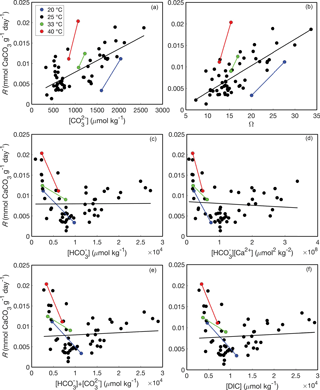Figure 1Aragonite precipitation rates as functions of fluid chemistry based on data from . Each point represents a separate abiogenic aragonite precipitation experiment conducted at 20 C (blue), 25 C (black), 33 C (green), and 40 C (red). Bulk aragonite precipitation rates (R) are plotted against mean fluid $\left[{\mathrm{CO}}_{\mathrm{3}}^{\mathrm{2}-}\right]$ (a), ΩAr (b), $\left[{\mathrm{HCO}}_{\mathrm{3}}^{-}\right]$ (c), [Ca2+]$\left[{\mathrm{HCO}}_{\mathrm{3}}^{-}\right]$ (d), $\left[{\mathrm{CO}}_{\mathrm{3}}^{\mathrm{2}-}\right]$+$\left[{\mathrm{HCO}}_{\mathrm{3}}^{-}\right]$ (e), and DIC (f). Solid lines show regression fits at each temperature (note that there are only two experiments at each temperature other than 25 C, and thus line fits for these temperatures should be interpreted with caution).

3 Fitting the experimental B∕Ca partitioning data

The second source of discrepancies between various applications of boron systematics to coral skeletons is the dependence of the KD on fluid chemistry. fit the KD as either a function of $\left[{\mathrm{CO}}_{\mathrm{3}}^{\mathrm{2}-}\right]$ or ΩAr, refit the data as a function of [H+], and fit data from both and as a function of ΩAr.

At the outset, it is important to recognize that there are two key differences between the abiogenic experiments of and . Firstly, precipitated aragonite from NaCl solutions, whereas used filtered seawater. Secondly, $\left[{\mathrm{CO}}_{\mathrm{3}}^{\mathrm{2}-}\right]$ and ΩAr are lower in the experiments of relative to . Potentially as result of one or both of these differences, found much lower KD values than . Here, we consider four possible KD dependencies based on these two experimental datasets (Fig. 2).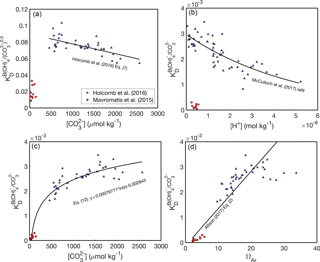Figure 2B∕Ca KD formulations. Abiogenic B∕Ca partitioning data from (red circles) and (blue triangles) fit as functions of fluid chemistry: $\left[{\mathrm{CO}}_{\mathrm{3}}^{\mathrm{2}-}\right]$ (a, c) , [H+] (b) , and ΩAr (d) (Allison2017). Note that KD in (a) is defined with Eq. (7) and in (b–d) is defined with Eq. (8). We use only the with [B] < 1000 µmol kg−1 due to the apparent effect of [B] on KD .

The first two formulations assume that there are substantial compositional effects on B∕Ca partitioning, and thus the offsets in KD between and arise due to the use of NaCl versus seawater solutions, respectively (Fig.  2a, b). If this is correct, the data are more appropriate for application to corals based on evidence that they precipitate their skeletons from seawater-based solutions . precipitated their aragonites from seawater solutions modified with [Ca2+] and [Mg2+] ranging between 6 and 20 and 48 and 98 mmol kg−1, respectively, without any apparent effects on the B∕Ca KD. While this suggests that the KD is not highly sensitive to seawater elemental chemistry, it is still possible that there are subtle compositional effects that have little influence on KD in modified seawater, but become apparent in the NaCl solutions used by . Assuming some compositional effects do exist, we are left with the two plausible KD expressions (Eqs. 7–8), and the previously presented dependencies on either $\left[{\mathrm{CO}}_{\mathrm{3}}^{\mathrm{2}-}\right]$ or [H+] .

Alternatively, it is possible that there are negligible effects from using NaCl or seawater solutions and, therefore, the data from both and should be fit by a single, continuous function. There are again two plausible formulations: KD increases as a function of $\left[{\mathrm{CO}}_{\mathrm{3}}^{\mathrm{2}-}\right]$ or ΩAr (Fig. 2c, d). Allison (2017) proposed a linear fit between KD and ΩAr that includes both the and data. From a practical standpoint, however, this latter approach is problematic in that it requires an independent proxy for [Ca2+] (see Sect. 8) and the linear fit effectively precludes its use for deriving coral calcifying fluid chemistry (see Sect. 4). In an attempt to avoid these issues, we introduce a logarithmic relationship between KD and $\left[{\mathrm{CO}}_{\mathrm{3}}^{\mathrm{2}-}\right]$, which fits both the and data (Fig. 2c):

$\begin{array}{ll}{K}_{D}^{\mathrm{B}/\mathrm{Ca}}& =\mathrm{0.00077}\left(±\mathrm{0.00007}\right)\cdot \mathrm{ln}\left(\left[{\mathrm{CO}}_{\mathrm{3}}^{\mathrm{2}-}\right]\right)\\ \text{(12)}& & -\mathrm{0.0028}\left(±\mathrm{0.0004}\right),\end{array}$

where parentheses indicate 95 % confidence, KD is defined by Eq. (8), and $\left[{\mathrm{CO}}_{\mathrm{3}}^{\mathrm{2}-}\right]$ is in units of micromoles per kilogram. Mechanistically, the increase in KD with $\left[{\mathrm{CO}}_{\mathrm{3}}^{\mathrm{2}-}\right]$ or ΩAr (or precipitation rate) is consistent with the surface entrapment model proposed by . In this model, minor element impurities, such as B, are incorporated in the near-surface layer of a growing crystal. Slower-growing crystals allow these impurities to diffuse out of the near-surface region into the fluid, whereas faster-growing crystals bury the near-surface impurities into the bulk crystal. The sensitivity of KD to $\left[{\mathrm{CO}}_{\mathrm{3}}^{\mathrm{2}-}\right]$ or ΩAr is also consistent with a surface kinetic model (DePaolo2011), in which trace element partitioning depends on the net rate of precipitation relative to dissolution. Thus, both the surface entrapment and kinetic models offer potential explanations as to why the low-ΩAr experiments of produced lower KD than the higher-ΩAr experiments of .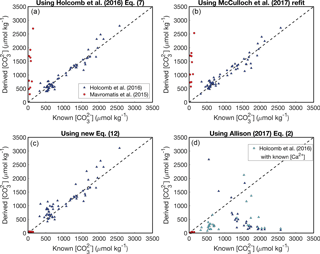Figure 3Reconstructing experimental fluid $\left[{\mathrm{CO}}_{\mathrm{3}}^{\mathrm{2}-}\right]$ using the KD formulations presented in Fig. 2. Symbols are the same as Fig. 2. In panel (d), negative $\left[{\mathrm{CO}}_{\mathrm{3}}^{\mathrm{2}-}\right]$ solutions have been excluded (see Appendix). Calculations using the KD formulation have been performed with both assuming seawater [Ca2+] (blue) and using the [Ca2+] reported from the experiments (cyan).

4 Back-application of partition coefficient formulations to abiogenic datasets

We conducted a simple test to evaluate the utility of the four KD dependencies considered above. For each KD formulation, we used the reported aragonite B∕Ca, fluid [B(OH)${}_{\mathrm{4}}^{-}$], and pH data of and to calculate the fluid $\left[{\mathrm{CO}}_{\mathrm{3}}^{\mathrm{2}-}\right]$, and then we compared the predicted $\left[{\mathrm{CO}}_{\mathrm{3}}^{\mathrm{2}-}\right]$ to the concentrations measured during the experiments (Fig. 3) (see also , for a similar analysis). The basis for this approach is to assess how well the experimental fluid $\left[{\mathrm{CO}}_{\mathrm{3}}^{\mathrm{2}-}\right]$ can be reconstructed using boron systematics alone. When boron systematics are applied to coral skeletons, $\left[{\mathrm{CO}}_{\mathrm{3}}^{\mathrm{2}-}\right]$ is predicted from only B∕Ca and δ11B. However, since δ11B was not reported by , we instead use the measured pH for the KD formulation. Additionally, since [B] was manipulated in some experiments, we use reported fluid [B(OH)${}_{\mathrm{4}}^{-}$] instead of calculating it from pH as is performed in applications to corals . Nevertheless, since pH (and thus seawater [B(OH)${}_{\mathrm{4}}^{-}$]) is readily calculated from δ11B, our approach is suitable for evaluating the utility of each KD formulation for reconstructing $\left[{\mathrm{CO}}_{\mathrm{3}}^{\mathrm{2}-}\right]$ with B∕Ca.

Since three of the KD formulations (, , and our new Eq. 12) themselves depend on $\left[{\mathrm{CO}}_{\mathrm{3}}^{\mathrm{2}-}\right]$, we solved for $\left[{\mathrm{CO}}_{\mathrm{3}}^{\mathrm{2}-}\right]$ as follows. An initial guess of $\left[{\mathrm{CO}}_{\mathrm{3}}^{\mathrm{2}-}\right]$ was used to calculate an initial KD, and this KD was used to solve for $\left[{\mathrm{CO}}_{\mathrm{3}}^{\mathrm{2}-}\right]$ by rearranging Eq. (7) to

$\begin{array}{}\text{(13)}& \left[{\mathrm{CO}}_{\mathrm{3}}^{\mathrm{2}-}\right]={\left({K}_{D}^{\mathrm{B}/\mathrm{Ca}}\frac{\left[\mathrm{B}\left(\mathrm{OH}{\right)}_{\mathrm{4}}^{-}{\right]}^{\mathrm{fluid}}}{\left[\mathrm{B}/\mathrm{Ca}{\right]}^{\mathrm{aragonite}}}\right)}^{\mathrm{2}}\end{array}$

and Eq. (8) to

$\begin{array}{}\text{(14)}& \left[{\mathrm{CO}}_{\mathrm{3}}^{\mathrm{2}-}\right]={K}_{D}^{\mathrm{B}/\mathrm{Ca}}\frac{\left[\mathrm{B}\left(\mathrm{OH}{\right)}_{\mathrm{4}}^{-}{\right]}^{\mathrm{fluid}}}{\left[\mathrm{B}/\mathrm{Ca}{\right]}^{\mathrm{aragonite}}},\end{array}$

where Eq. (14) is used for and our new Eq. (12), and Eq. (13) is used for . We then calculated the residual between the calculated (Eqs. 13–14) and initially estimated $\left[{\mathrm{CO}}_{\mathrm{3}}^{\mathrm{2}-}\right]$. Finally, we iteratively adjusted the initial $\left[{\mathrm{CO}}_{\mathrm{3}}^{\mathrm{2}-}\right]$ estimate for each data point until it equaled the $\left[{\mathrm{CO}}_{\mathrm{3}}^{\mathrm{2}-}\right]$ derived from Eqs. (13)–(14).

Both the fit (their Eq. 7) and the refit perform similarly, effectively reconstructing the fluid $\left[{\mathrm{CO}}_{\mathrm{3}}^{\mathrm{2}-}\right]$ of the experimental data (root mean square error, RMSE = 151 and 163 µmol kg−1, respectively), but performing poorly for the data (RMSE = 1370 and 1385 µmol kg−1, respectively) (Fig. 3a, b). This is not surprising because these KD dependencies are offset from the data (Fig. 2a, b). Our new logarithmic equation performs well for both datasets (RMSE = 42 and 204 µmol kg−1 for and , respectively). The formulation (assuming [Ca2+] of 10 µmol kg−1) performs well for the data (RMSE = 51 µmol kg−1), but creates a trend opposite that expected for the data (RMSE = 1375 µmol kg−1) (Fig. 3d). Using the reported [Ca2+] and Ksp from the experiments in the formulation improves the results slightly and generates more positive solutions, but the RMSE is still 950 µmol kg−1.

An alternative way to understand these patterns is to investigate the relationship between $\left[{\mathrm{CO}}_{\mathrm{3}}^{\mathrm{2}-}\right]$ and the ratio of fluid [B(OH)${}_{\mathrm{4}}^{-}$] to solid B∕Ca (Fig. 4). Following Eqs. (13)–(14), $\left[{\mathrm{CO}}_{\mathrm{3}}^{\mathrm{2}-}\right]$ should be positively related to $\frac{\left[\mathrm{B}\left(\mathrm{OH}{\right)}_{\mathrm{4}}^{-}{\right]}^{\mathrm{fluid}}}{\left[\mathrm{B}/\mathrm{Ca}{\right]}^{\mathrm{aragonite}}}$, and this behavior is clearly evident in the abiogenic aragonites of (blue triangles in Fig. 4). The KD formulations of , , and our new Eq. (12) all closely track the abiogenic data, especially for $\left[{\mathrm{CO}}_{\mathrm{3}}^{\mathrm{2}-}\right]$< 2000 µmol kg−1. Conversely, the fit (assuming [Ca2+] of 10 mmol kg−1) produces the opposite trend and is invalid or negative below a $\frac{\left[\mathrm{B}\left(\mathrm{OH}{\right)}_{\mathrm{4}}^{-}{\right]}^{\mathrm{fluid}}}{\left[\mathrm{B}/\mathrm{Ca}{\right]}^{\mathrm{aragonite}}}$ of  0.44 mol kg−1 (see Appendix for derivation of an analytical solution).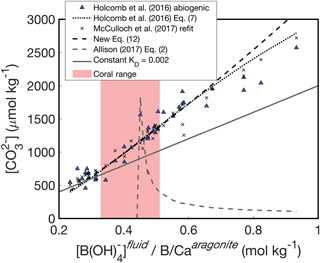Figure 4Experimental fluid $\left[{\mathrm{CO}}_{\mathrm{3}}^{\mathrm{2}-}\right]$ as a function of $\frac{\left[\mathrm{B}\left(\mathrm{OH}{\right)}_{\mathrm{4}}^{-}{\right]}^{\mathrm{fluid}}}{\left[\mathrm{B}/\mathrm{Ca}{\right]}^{\mathrm{aragonite}}}$. The KD formulations of (dotted black line), (black crosses), and Eq. (12) (dashed black line) all capture the trend of increasing $\left[{\mathrm{CO}}_{\mathrm{3}}^{\mathrm{2}-}\right]$ with increasing $\frac{\left[\mathrm{B}\left(\mathrm{OH}{\right)}_{\mathrm{4}}^{-}{\right]}^{\mathrm{fluid}}}{\left[\mathrm{B}/\mathrm{Ca}{\right]}^{\mathrm{aragonite}}}$ that is apparent in the abiogenic data (blue triangles). A constant KD (solid grey line) underestimates the slope between $\frac{\left[\mathrm{B}\left(\mathrm{OH}{\right)}_{\mathrm{4}}^{-}{\right]}^{\mathrm{fluid}}}{\left[\mathrm{B}/\mathrm{Ca}{\right]}^{\mathrm{aragonite}}}$ and $\left[{\mathrm{CO}}_{\mathrm{3}}^{\mathrm{2}-}\right]$. The pink shaded region shows the range of $\frac{\left[\mathrm{B}\left(\mathrm{OH}{\right)}_{\mathrm{4}}^{-}{\right]}^{\mathrm{fluid}}}{\left[\mathrm{B}/\mathrm{Ca}{\right]}^{\mathrm{aragonite}}}$ derived for Porites corals by .

The behavior of the KD formulations can be understood by inspecting the residuals between initial $\left[{\mathrm{CO}}_{\mathrm{3}}^{\mathrm{2}-}\right]$ estimates and those derived from Eqs. (13)–(14) (Fig. 5). The KD formulation generates unique $\left[{\mathrm{CO}}_{\mathrm{3}}^{\mathrm{2}-}\right]$ solutions (i.e., where the residual equals zero) that increase with $\frac{\left[\mathrm{B}\left(\mathrm{OH}{\right)}_{\mathrm{4}}^{-}{\right]}^{\mathrm{fluid}}}{\left[\mathrm{B}/\mathrm{Ca}{\right]}^{\mathrm{aragonite}}}$ (Fig. 5a), which is the ideal behavior. Our new Eq. (12) also produces increasing $\left[{\mathrm{CO}}_{\mathrm{3}}^{\mathrm{2}-}\right]$ solutions with increasing $\frac{\left[\mathrm{B}\left(\mathrm{OH}{\right)}_{\mathrm{4}}^{-}{\right]}^{\mathrm{fluid}}}{\left[\mathrm{B}/\mathrm{Ca}{\right]}^{\mathrm{aragonite}}}$ (Fig. 5b); however, a major issue of this formulation is that there may be two $\left[{\mathrm{CO}}_{\mathrm{3}}^{\mathrm{2}-}\right]$ solutions for each $\frac{\left[\mathrm{B}\left(\mathrm{OH}{\right)}_{\mathrm{4}}^{-}{\right]}^{\mathrm{fluid}}}{\left[\mathrm{B}/\mathrm{Ca}{\right]}^{\mathrm{aragonite}}}$. Finally, although the KD formulation produces unique $\left[{\mathrm{CO}}_{\mathrm{3}}^{\mathrm{2}-}\right]$ solutions, they increase with decreasing $\frac{\left[\mathrm{B}\left(\mathrm{OH}{\right)}_{\mathrm{4}}^{-}{\right]}^{\mathrm{fluid}}}{\left[\mathrm{B}/\mathrm{Ca}{\right]}^{\mathrm{aragonite}}}$ (Fig. 5c), opposite to that expected (Fig. 4).

The reason for the poor behavior of the formulation is the linear fit between KD and ΩAr with an intercept near the origin. When using this formulation to predict $\left[{\mathrm{CO}}_{\mathrm{3}}^{\mathrm{2}-}\right]$ from boron systematics alone, we must assume [Ca2+] is approximately equal to seawater (10 mmol kg−1), meaning that ΩAr is directly related to $\left[{\mathrm{CO}}_{\mathrm{3}}^{\mathrm{2}-}\right]$. Since the intercept in the KD formulation is close to the origin, any change in $\left[{\mathrm{CO}}_{\mathrm{3}}^{\mathrm{2}-}\right]$ results in an almost proportional change in KD. It can be seen why this is problematic by inspecting how $\left[{\mathrm{CO}}_{\mathrm{3}}^{\mathrm{2}-}\right]$ is derived from Eq. (14). The $\frac{\left[\mathrm{B}\left(\mathrm{OH}{\right)}_{\mathrm{4}}^{-}{\right]}^{\mathrm{fluid}}}{\left[\mathrm{B}/\mathrm{Ca}{\right]}^{\mathrm{aragonite}}}$ is derived from pH (or δ11B) and measured B∕Ca, so this ratio remains constant while we find the appropriate KD that minimizes the residual $\left[{\mathrm{CO}}_{\mathrm{3}}^{\mathrm{2}-}\right]$, as in Fig. 5. Therefore, Eq. (14) is effectively reduced to $\left[{\mathrm{CO}}_{\mathrm{3}}^{\mathrm{2}-}\right]$ being a function of KD multiplied by a constant. However, since KD changes almost directly proportionally to $\left[{\mathrm{CO}}_{\mathrm{3}}^{\mathrm{2}-}\right]$ according to , it is difficult to find a $\left[{\mathrm{CO}}_{\mathrm{3}}^{\mathrm{2}-}\right]$ that explains different $\frac{\left[\mathrm{B}\left(\mathrm{OH}{\right)}_{\mathrm{4}}^{-}{\right]}^{\mathrm{fluid}}}{\left[\mathrm{B}/\mathrm{Ca}{\right]}^{\mathrm{aragonite}}}$ values. Although recognized the difficulty of explaining the range of B∕Ca observed in corals (see their Fig. 8g), the implication of applying this KD formulation to predict $\left[{\mathrm{CO}}_{\mathrm{3}}^{\mathrm{2}-}\right]$ was not discussed. Our analysis suggests that this KD formulation is poorly suited for accurately reconstructing fluid $\left[{\mathrm{CO}}_{\mathrm{3}}^{\mathrm{2}-}\right]$ from boron systematics (Figs. 3d, 4).

Another approach presented by is to use a constant KD. We selected a KD value of 0.02 as an example that fits the abiogenic data near the low-$\left[{\mathrm{CO}}_{\mathrm{3}}^{\mathrm{2}-}\right]$ range of the data (Fig. 4). While a constant KD performs better than the linear fit to ΩAr, it underestimates the slope of the relationship between $\frac{\left[\mathrm{B}\left(\mathrm{OH}{\right)}_{\mathrm{4}}^{-}{\right]}^{\mathrm{fluid}}}{\left[\mathrm{B}/\mathrm{Ca}{\right]}^{\mathrm{aragonite}}}$ and $\left[{\mathrm{CO}}_{\mathrm{3}}^{\mathrm{2}-}\right]$ (Fig. 4). This is not surprising because the abiogenic data clearly show the KD does not remain constant as $\left[{\mathrm{CO}}_{\mathrm{3}}^{\mathrm{2}-}\right]$ changes (Fig. 2). Since using a constant KD will underestimate variability in $\left[{\mathrm{CO}}_{\mathrm{3}}^{\mathrm{2}-}\right]$cf when applied to corals, we do not recommend this approach.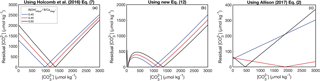Figure 5Predicting $\left[{\mathrm{CO}}_{\mathrm{3}}^{\mathrm{2}-}\right]$ from the KD formulations which themselves depend on $\left[{\mathrm{CO}}_{\mathrm{3}}^{\mathrm{2}-}\right]$: (a), Eq. (12) (b), and (c). Each panel shows the residual between a guess of $\left[{\mathrm{CO}}_{\mathrm{3}}^{\mathrm{2}-}\right]$ used to calculate KD and that calculated from Eqs. (13)–(14), plotted against the $\left[{\mathrm{CO}}_{\mathrm{3}}^{\mathrm{2}-}\right]$ guess. The final $\left[{\mathrm{CO}}_{\mathrm{3}}^{\mathrm{2}-}\right]$ is derived by finding where the residual is minimized for a particular $\frac{\left[\mathrm{B}\left(\mathrm{OH}{\right)}_{\mathrm{4}}^{-}{\right]}^{\mathrm{fluid}}}{\left[\mathrm{B}/\mathrm{Ca}{\right]}^{\mathrm{aragonite}}}$ (three of which are plotted as examples in red, blue, and black).

5 Application to deriving coral calcifying fluid carbonate chemistry

The ability of boron systematics to predict two independent carbonate chemistry parameters allows for calculation of the full carbonate system. This has prompted several recent applications deriving the carbonate chemistry of coral calcifying fluids (; Comeau et al., 2017a; ; ; ; ; ). Here, we investigate the differences in derived coral calcifying fluid $\left[{\mathrm{CO}}_{\mathrm{3}}^{\mathrm{2}-}\right]$ that arise from the choice of KD formulation. We use the paired δ11B and B∕Ca data of the “Davies 2” coral from as an example.

Derived $\left[{\mathrm{CO}}_{\mathrm{3}}^{\mathrm{2}-}\right]$cf shows similar seasonality when using the KD formulations of , , or our new Eq. (12) (Fig. 6). Regardless of which of these three KD formulations are used, $\left[{\mathrm{CO}}_{\mathrm{3}}^{\mathrm{2}-}\right]$cf is highest in summer and lowest in winter over a multiyear time series. This is consistent with other reports of B∕Ca seasonality in coral skeletons , and with an independent approach based on Rayleigh modeling of minor elements in coral skeleton . The primary difference among the derived values is that the KD formulations from and our Eq. (12) produce seasonal cycles with  50 % greater amplitude relative to the KD formulation. The absolute values of derived $\left[{\mathrm{CO}}_{\mathrm{3}}^{\mathrm{2}-}\right]$cf is approximately equal for all three formulations at the summertime maxima, but are lower during winter when using the KD formulations from or our Eq. (12), relative to . Conversely, using the KD formulation produces the opposite seasonal pattern with amplitude several times greater than the other KD formulations. This large discrepancy is not surprising given the behavior of the KD formulation when retrospectively applied to the fluid composition of abiogenic aragonites (Fig. 3).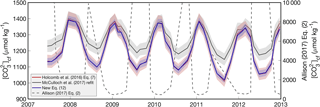Figure 6Application of the four KD formulations for the “Davies 2” Porites coral data from . Derived $\left[{\mathrm{CO}}_{\mathrm{3}}^{\mathrm{2}-}\right]$cf is plotted over multiple years using the KD formulations of (black), (red), Eq. (12) (blue), and (dashed grey line). Shading represents 1 standard deviation of the systematic errors due to uncertainty in each KD formulation. Note that (1) the and the Eq. (12) lines plot nearly on top of each other, and (2) $\left[{\mathrm{CO}}_{\mathrm{3}}^{\mathrm{2}-}\right]$cf derived from the KD formulation corresponds to the right y axis.

6 A computer code for applying boron systematics to coral skeletons

We present here a user-friendly computer code for deriving $\left[{\mathrm{CO}}_{\mathrm{3}}^{\mathrm{2}-}\right]$cf and DICcf from boron systematics (Supplement). The function is provided in both MATLAB and R formats, and it calculates $\left[{\mathrm{CO}}_{\mathrm{3}}^{\mathrm{2}-}\right]$cf and DICcf given inputs of δ11B, B∕Ca, temperature, salinity, and water depth. It allows easy toggling between what we consider the three plausible KD formulations (, , and our new Eq. 12). Furthermore, the code permits a choice of [B]sw functions since and used the relation between salinity and [B]sw from , whereas and used that of . The carbonate dissociation constants can also be toggled between and . The code follows the calculations of CO2SYS for converting between pH scales and accounting for pressure effects on equilibrium constants, and uses the δ11Bsw of and the αB3−B4 of .

Perhaps most importantly, the code propagates known uncertainties into the derivation of $\left[{\mathrm{CO}}_{\mathrm{3}}^{\mathrm{2}-}\right]$cf and DICcf. These uncertainties are estimated using a Monte Carlo scheme, in which random errors (assuming Gaussian distributions) are added to parameters while repeating the calculations many times. The non-systematic uncertainty of derived values depends on the measurement precisions of δ11B, B∕Ca, temperature, and salinity. These will depend on the instruments and protocols used, and for δ11B and B∕Ca should be estimated by each laboratory, for example by repeated measurements of an external consistency standard. The systemic errors of derived values depend on the uncertainties of the various KD formulations, uncertainties associated with δ11Bsw , [B]sw , αB3−B4 , and pKB (Dickson1990), and if known, any uncertainties in the accuracy of δ11B, B∕Ca, temperature, and salinity measurements.

7 Relationships among coral calcifying fluid carbonate chemistry parameters

With our code, the parameter space of $\left[{\mathrm{CO}}_{\mathrm{3}}^{\mathrm{2}-}\right]$cf derived from δ11B and B∕Ca, and the differences among KD formulations, can be readily visualized (Fig. 7). This enables future applications of boron systematics to coral skeletons to consider how the choice of KD formulation affects the particular question being investigated. We also apply the code to calculate carbonate system parameters using published δ11B and B∕Ca datasets (Fig. 8). Coral δ11B is tightly related to pHcf, varying only slightly with changes in seawater temperature and salinity (Fig. 8b). Likewise, B∕Ca is primarily a function of $\left[{\mathrm{CO}}_{\mathrm{3}}^{\mathrm{2}-}\right]$cf, but also depends in part on borate concentration, and hence on pHcf (Fig. 8c). For this reason, it is difficult to directly interpret coral B∕Ca, and instead we recommend pairing δ11B and B∕Ca to calculate the full calcifying fluid carbonate chemistry.

Interestingly, this analysis shows that coral calcifying fluid $\left[{\mathrm{CO}}_{\mathrm{3}}^{\mathrm{2}-}\right]$cf and DIC are consistently positively correlated across studies (Fig. 8f), whereas the sign of correlations between pH and both $\left[{\mathrm{CO}}_{\mathrm{3}}^{\mathrm{2}-}\right]$cf and DIC varies (Fig. 8d–e). Assuming $\left[{\mathrm{CO}}_{\mathrm{3}}^{\mathrm{2}-}\right]$cf is the carbonate system parameter most important for aragonite precipitation, these patterns may suggest that elevating DICcf is critical to the coral calcification process, although up-regulating pH is still important for shifting the carbonate system to favor ${\mathrm{CO}}_{\mathrm{3}}^{\mathrm{2}-}$ over HCO${}_{\mathrm{3}}^{-}$. In addition, the large changes in pH, DIC, and $\left[{\mathrm{CO}}_{\mathrm{3}}^{\mathrm{2}-}\right]$ that occur within the calcifying fluid relative to natural variability in the open ocean likely preclude the utility of boron systematics for reconstructing seawater carbonate chemistry, reinforcing previous conclusions made for both corals and foraminifera . Rather, the boron systematics of coral skeletons are primarily useful for investigating calcifying fluid dynamics and understanding coral biomineralization.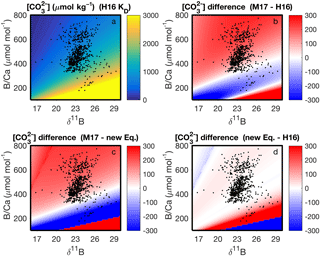Figure 7Application of our computer code to visualizing the parameter space of $\left[{\mathrm{CO}}_{\mathrm{3}}^{\mathrm{2}-}\right]$ (µmol kg−1) derived from B∕Ca and δ11B at 25 C and salinity 35. The upper left panel shows absolute $\left[{\mathrm{CO}}_{\mathrm{3}}^{\mathrm{2}-}\right]$ derived with the KD of (“H16”), whereas the other panels show the differences in $\left[{\mathrm{CO}}_{\mathrm{3}}^{\mathrm{2}-}\right]$ among the KD formulations of H16, (“M17”), and our new Eq. (12). The black dots show coral data from the literature (see Fig. 8 legend below). Note that the actual $\left[{\mathrm{CO}}_{\mathrm{3}}^{\mathrm{2}-}\right]$ derived for the coral data will also depend on variations of the in situ temperature and salinity, which are not accounted for in the plots.

In contrast to boron systemics, which consistently show elevated DICcf, microelectrode measurements of $\left[{\mathrm{CO}}_{\mathrm{3}}^{\mathrm{2}-}\right]$cf and pHcf imply that DICcf is depleted , relative to that of seawater (typically $<\mathrm{2000}\phantom{\rule{0.125em}{0ex}}\mathrm{µ}\mathrm{mol}\phantom{\rule{0.125em}{0ex}}{\mathrm{kg}}^{-\mathrm{1}}$). The reason for this discrepancy is not yet clear, and resolving it should be a high priority because whether DICcf is greater than or less than seawater implies different calcification strategies. Reducing DICcf may be an efficient strategy to increase pHcf because the reduced buffering capacity means that less energy is required to elevate pH via proton pumping . Alternatively, increasing DICcf means that a higher ΩAr is achieved for a given pHcf. Deciphering between these possibilities has key implications for whether calcification is limited by DICcf (or CO2 diffusion into the calcifying fluid) or by ΩAr. Two independent approaches to quantifying calcifying fluid carbonate chemistry are consistent with the high DICcf scenario. First, coral U∕Ca ratios imply that DICcf is between 2600 and 6100 µmol kg−1 , which is a similar range to that derived from boron systematics (Fig. 8). Second, boron systematics-derived $\left[{\mathrm{CO}}_{\mathrm{3}}^{\mathrm{2}-}\right]$ is consistent with a combination of Raman-spectroscopy-derived ΩAr and trace element ratios (Mg / Ca and Sr / Ca) . Nevertheless, since low DICcf has been derived from microelectrodes in several species , studies combining multiple approaches (i.e., geochemistry and microelectrodes) on the same specimens will be essential for resolving the DICcf discrepancy.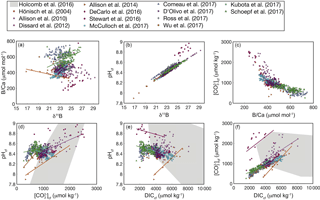Figure 8Correlations among coral calcifying fluid carbonate system parameters based on published boron systematics datasets: (a) B∕Ca and δ11B, (b) pHcf and δ11B, (c) $\left[{\mathrm{CO}}_{\mathrm{3}}^{\mathrm{2}-}\right]$cf and B∕Ca, (d) pH and $\left[{\mathrm{CO}}_{\mathrm{3}}^{\mathrm{2}-}\right]$cf, (e) pHcf and DICcf, and (f) $\left[{\mathrm{CO}}_{\mathrm{3}}^{\mathrm{2}-}\right]$cf and DICcf. Colors show different studies, and lines are plotted for significant (p<0.05) correlations using all the data within each study. The grey area shows the convex hull of the parameter space covered in the abiogenic experiments of . Calculations are performed using the KD formulation.

8 Which KD formulation to use?

Despite the availability of abiogenic B∕Ca partitioning data from two experiments , and several attempts to fit the data , it is important to recognize that uncertainties still remain, in particular an understanding of the controlling factors, and thus the appropriate KD formulation to apply. From a mechanistic viewpoint, the key fundamental question that remains is whether the abiogenic data of and are directly comparable and thus should be fit with a continuous function (e.g., Eq. 12), or if they are incomparable because used NaCl solutions and used seawater. If they are comparable, then our new Eq. (12) or a similar fit to both datasets is the most appropriate KD formulation. Calcite precipitation studies provide some support for the hypothesis that crystal growth rate or ΩAr influences B∕Ca partitioning , but it is not yet known if these results can be extended to aragonite precipitation from seawater. Alternatively, if the solution chemistry makes the two experiments incomparable, the KD data are most likely the more suitable choice for corals because the experiments were conducted with seawater at ΩAr comparable to that of coral calcifying fluids , and they can be fit as a function of either $\left[{\mathrm{CO}}_{\mathrm{3}}^{\mathrm{2}-}\right]$ or [H+]. However, it is important to recognize that the parameter space of CO2 system parameters covered in the experiments includes some, but not all, of the published coral data (Fig. 8). Further, since we are unable to conclusively distinguish whether the two abiogenic datasets are directly comparable, all three KD formulations may be considered equally valid until proven otherwise. Additional abiogenic experiments aimed at this question will clearly be useful in refining the boron systematics proxies.

From a practical standpoint, the KD formulations of and may be the most appropriate. Both produce unique solutions of $\left[{\mathrm{CO}}_{\mathrm{3}}^{\mathrm{2}-}\right]$ that increase with $\frac{\left[\mathrm{B}\left(\mathrm{OH}{\right)}_{\mathrm{4}}^{-}{\right]}^{\mathrm{fluid}}}{\left[\mathrm{B}/\mathrm{Ca}{\right]}^{\mathrm{aragonite}}}$, and they effectively reconstruct fluid $\left[{\mathrm{CO}}_{\mathrm{3}}^{\mathrm{2}-}\right]$ using the abiogenic aragonites precipitated from seawater. While our Eq. (12) produces $\left[{\mathrm{CO}}_{\mathrm{3}}^{\mathrm{2}-}\right]$cf estimates that are nearly identical under most δ11B and B∕Ca combinations to those derived using the KD formulation (Fig. 7), Eq. (12) can have nonunique solutions, which could complicate interpretations of $\left[{\mathrm{CO}}_{\mathrm{3}}^{\mathrm{2}-}\right]$cf in some cases.

A final consideration is that two of the KD formulations ( and our new Eq. 12) are fit to $\left[{\mathrm{CO}}_{\mathrm{3}}^{\mathrm{2}-}\right]$. Fitting Eq. (12) to a wider range of $\left[{\mathrm{CO}}_{\mathrm{3}}^{\mathrm{2}-}\right]$ helps to account for the different solution chemistries and associated growth rates of the two abiogenic precipitation studies , but ΩAr or crystal growth rate may be the true controlling factor . However, did not find a temperature dependence of B∕Ca partitioning, as would be expected if precipitation rate influenced KD. While growth rate is likely related to $\left[{\mathrm{CO}}_{\mathrm{3}}^{\mathrm{2}-}\right]$ , the two could decouple with changes in temperature or if coral calcifying fluid [Ca2+]cf departs from seawater levels. Recent evidence combining Raman spectroscopy with boron systematics suggests [Ca2+]cf is within  25 % of seawater (; DeCarlo et al., 2018) but this has yet to be tested on a range of coral species and locations. Thus, future abiogenic experiments designed to test under what conditions $\left[{\mathrm{CO}}_{\mathrm{3}}^{\mathrm{2}-}\right]$ or crystal growth rates control B∕Ca partitioning, as well as development of proxies for [Ca2+]cf, may improve the accuracy of deriving calcifying fluid carbonate chemistry from boron systematics.

9 Conclusions

Recent abiogenic aragonite precipitation experiments have made possible the application of boron systematics to quantifying the full carbonate system of coral calcifying fluid. However, a number of approaches to doing so have been utilized (; ; ), without a comprehensive analysis of which KD formulations are plausible (i.e., can reproduce the experimental fluid chemistry) or the implications for interpreting coral skeletons. We evaluated four potential B∕Ca KD formulations involving B(OH)${}_{\mathrm{4}}^{-}$ substituting for ${\mathrm{CO}}_{\mathrm{3}}^{\mathrm{2}-}$ in the aragonite lattice. Our analysis suggests that there are at least three plausible KD formulations (, , and our new Eq. 12) that can be used to determine the KD and its dependence on fluid chemistry. Despite the differences in plausible approaches, we show that all three produce similar patterns in derived coral calcifying fluid carbonate chemistry. Nevertheless, subtle differences in derived carbonate chemistry remain among the approaches, and addressing these differences should be the target of future abiogenic aragonite precipitation experiments. Finally, we present a code that computes coral calcifying fluid carbonate chemistry from boron systematics and allows for comparison among different KD formulations.

Code availability

Codes are available in the Supplement.

Appendix A

In the main text, we used numerical solutions to predict $\left[{\mathrm{CO}}_{\mathrm{3}}^{\mathrm{2}-}\right]$ based on Eq. (14). Here, we show an analytical solution to Eq. (14) for the KD formulation. fit KD to ΩAr with a linear regression in the form:

$\begin{array}{}\text{(A1)}& {K}_{D}^{\mathrm{B}/\mathrm{Ca}}=a\mathrm{\Omega }+b,\end{array}$

where

$\begin{array}{}\text{(A2)}& \mathrm{\Omega }=\frac{\left[{\mathrm{CO}}_{\mathrm{3}}^{\mathrm{2}-}\right]\left[{\mathrm{Ca}}^{\mathrm{2}+}\right]}{{K}_{\mathrm{sp}}},\end{array}$

with concentrations in units of moles per kilogram and where Ksp is the solubility product. Inserting Eq. (A2) into Eq. (A1) yields

$\begin{array}{}\text{(A3)}& {K}_{D}^{\mathrm{B}/\mathrm{Ca}}=a\frac{\left[{\mathrm{CO}}_{\mathrm{3}}^{\mathrm{2}-}\right]\left[{\mathrm{Ca}}^{\mathrm{2}+}\right]}{{K}_{\mathrm{sp}}}+b,\end{array}$

and then inserting Eq. (A3) into Eq. (14) of the main text yields

$\begin{array}{}\text{(A4)}& \left[{\mathrm{CO}}_{\mathrm{3}}^{\mathrm{2}-}\right]=\left(a\frac{\left[{\mathrm{CO}}_{\mathrm{3}}^{\mathrm{2}-}\right]\left[{\mathrm{Ca}}^{\mathrm{2}+}\right]}{{K}_{\mathrm{sp}}}+b\right)\frac{\left[\mathrm{B}\left(\mathrm{OH}{\right)}_{\mathrm{4}}^{-}{\right]}^{\mathrm{fluid}}}{\left[\mathrm{B}/\mathrm{Ca}{\right]}^{\mathrm{aragonite}}},\end{array}$

where $\left[{\mathrm{CO}}_{\mathrm{3}}^{\mathrm{2}-}\right]$ is in units of moles per kilogram. We must now solve Eq. (A4) for $\left[{\mathrm{CO}}_{\mathrm{3}}^{\mathrm{2}-}\right]$. First, expand the right side of the equation:

$\begin{array}{ll}\left[{\mathrm{CO}}_{\mathrm{3}}^{\mathrm{2}-}\right]& =a\frac{\left[{\mathrm{CO}}_{\mathrm{3}}^{\mathrm{2}-}\right]\left[{\mathrm{Ca}}^{\mathrm{2}+}\right]}{{K}_{\mathrm{sp}}}\frac{\left[\mathrm{B}\left(\mathrm{OH}{\right)}_{\mathrm{4}}^{-}{\right]}^{\mathrm{fluid}}}{\left[\mathrm{B}/\mathrm{Ca}{\right]}^{\mathrm{aragonite}}}\\ \text{(A5)}& & +b\frac{\left[\mathrm{B}\left(\mathrm{OH}{\right)}_{\mathrm{4}}^{-}{\right]}^{\mathrm{fluid}}}{\left[\mathrm{B}/\mathrm{Ca}{\right]}^{\mathrm{aragonite}}}.\end{array}$

Multiply both sides by Ksp:

$\begin{array}{ll}\left[{\mathrm{CO}}_{\mathrm{3}}^{\mathrm{2}-}\right]{K}_{\mathrm{sp}}& =a\left[{\mathrm{CO}}_{\mathrm{3}}^{\mathrm{2}-}\right]\left[{\mathrm{Ca}}^{\mathrm{2}+}\right]\frac{\left[\mathrm{B}\left(\mathrm{OH}{\right)}_{\mathrm{4}}^{-}{\right]}^{\mathrm{fluid}}}{\left[\mathrm{B}/\mathrm{Ca}{\right]}^{\mathrm{aragonite}}}\\ \text{(A6)}& & +b\frac{\left[\mathrm{B}\left(\mathrm{OH}{\right)}_{\mathrm{4}}^{-}{\right]}^{\mathrm{fluid}}}{\left[\mathrm{B}/\mathrm{Ca}{\right]}^{\mathrm{aragonite}}}{K}_{\mathrm{sp}}.\end{array}$

Collect all the $\left[{\mathrm{CO}}_{\mathrm{3}}^{\mathrm{2}-}\right]$ terms on the left side of the equation:

$\begin{array}{ll}\left[{\mathrm{CO}}_{\mathrm{3}}^{\mathrm{2}-}\right]{K}_{\mathrm{sp}}& -a\left[{\mathrm{CO}}_{\mathrm{3}}^{\mathrm{2}-}\right]\left[{\mathrm{Ca}}^{\mathrm{2}+}\right]\frac{\left[\mathrm{B}\left(\mathrm{OH}{\right)}_{\mathrm{4}}^{-}{\right]}^{\mathrm{fluid}}}{\left[\mathrm{B}/\mathrm{Ca}{\right]}^{\mathrm{aragonite}}}\\ \text{(A7)}& & =b\frac{\left[\mathrm{B}\left(\mathrm{OH}{\right)}_{\mathrm{4}}^{-}{\right]}^{\mathrm{fluid}}}{\left[\mathrm{B}/\mathrm{Ca}{\right]}^{\mathrm{aragonite}}}{K}_{\mathrm{sp}}.\end{array}$

Factor out $\left[{\mathrm{CO}}_{\mathrm{3}}^{\mathrm{2}-}\right]$:

$\begin{array}{ll}\left[{\mathrm{CO}}_{\mathrm{3}}^{\mathrm{2}-}\right]& \left({K}_{\mathrm{sp}}-a\left[{\mathrm{Ca}}^{\mathrm{2}+}\right]\frac{\left[\mathrm{B}\left(\mathrm{OH}{\right)}_{\mathrm{4}}^{-}{\right]}^{\mathrm{fluid}}}{\left[\mathrm{B}/\mathrm{Ca}{\right]}^{\mathrm{aragonite}}}\right)\\ \text{(A8)}& & =b\frac{\left[\mathrm{B}\left(\mathrm{OH}{\right)}_{\mathrm{4}}^{-}{\right]}^{\mathrm{fluid}}}{\left[\mathrm{B}/\mathrm{Ca}{\right]}^{\mathrm{aragonite}}}{K}_{\mathrm{sp}}.\end{array}$

Solve for $\left[{\mathrm{CO}}_{\mathrm{3}}^{\mathrm{2}-}\right]$:

$\begin{array}{}\text{(A9)}& \left[{\mathrm{CO}}_{\mathrm{3}}^{\mathrm{2}-}\right]=\frac{b\frac{\left[\mathrm{B}\left(\mathrm{OH}{\right)}_{\mathrm{4}}^{-}{\right]}^{\mathrm{fluid}}}{\left[\mathrm{B}/\mathrm{Ca}{\right]}^{\mathrm{aragonite}}}{K}_{\mathrm{sp}}}{{K}_{\mathrm{sp}}-a\left[{\mathrm{Ca}}^{\mathrm{2}+}\right]\frac{\left[\mathrm{B}\left(\mathrm{OH}{\right)}_{\mathrm{4}}^{-}{\right]}^{\mathrm{fluid}}}{\left[\mathrm{B}/\mathrm{Ca}{\right]}^{\mathrm{aragonite}}}}.\end{array}$

In seawater at 25 C and salinity 34, [Ca2+] is approximately 0.01 mol kg−1 and Ksp is $\mathrm{6.54}×{\mathrm{10}}^{-\mathrm{7}}$ . According to , a is $\mathrm{1.48}×{\mathrm{10}}^{-\mathrm{4}}$ and b is $-\mathrm{1.30}×{\mathrm{10}}^{-\mathrm{4}}$. Inserting these values in Eq. (A9) yields

$\begin{array}{ll}\left[{\mathrm{CO}}_{\mathrm{3}}^{\mathrm{2}-}\right]& =\frac{\left(-\mathrm{1.30}×{\mathrm{10}}^{-\mathrm{4}}\right)\frac{\left[\mathrm{B}\left(\mathrm{OH}{\right)}_{\mathrm{4}}^{-}{\right]}^{\mathrm{fluid}}}{\left[\mathrm{B}/\mathrm{Ca}{\right]}^{\mathrm{aragonite}}}\left(\mathrm{6.54}×{\mathrm{10}}^{-\mathrm{7}}\right)}{\left(\mathrm{6.54}×{\mathrm{10}}^{-\mathrm{7}}\right)-\left(\mathrm{1.48}×{\mathrm{10}}^{-\mathrm{4}}\right)\left(\mathrm{0.01}\right)\frac{\left[\mathrm{B}\left(\mathrm{OH}{\right)}_{\mathrm{4}}^{-}{\right]}^{\mathrm{fluid}}}{\left[\mathrm{B}/\mathrm{Ca}{\right]}^{\mathrm{aragonite}}}}\\ \text{(A10)}& & =\frac{-\mathrm{8.50}×{\mathrm{10}}^{-\mathrm{11}}\frac{\left[\mathrm{B}\left(\mathrm{OH}{\right)}_{\mathrm{4}}^{-}{\right]}^{\mathrm{fluid}}}{\left[\mathrm{B}/\mathrm{Ca}{\right]}^{\mathrm{aragonite}}}}{\mathrm{6.54}×{\mathrm{10}}^{-\mathrm{7}}-\mathrm{1.48}x{\mathrm{10}}^{-\mathrm{6}}\frac{\left[\mathrm{B}\left(\mathrm{OH}{\right)}_{\mathrm{4}}^{-}{\right]}^{\mathrm{fluid}}}{\left[\mathrm{B}/\mathrm{Ca}{\right]}^{\mathrm{aragonite}}}}.\end{array}$

The denominator equals zero (i.e., the solution is undefined) when $\frac{\left[\mathrm{B}\left(\mathrm{OH}{\right)}_{\mathrm{4}}^{-}{\right]}^{\mathrm{fluid}}}{\left[\mathrm{B}/\mathrm{Ca}{\right]}^{\mathrm{aragonite}}}$ = $\frac{\mathrm{6.54}×{\mathrm{10}}^{-\mathrm{7}}}{\mathrm{1.48}×{\mathrm{10}}^{-\mathrm{6}}}$= 0.44. If $\frac{\left[\mathrm{B}\left(\mathrm{OH}{\right)}_{\mathrm{4}}^{-}{\right]}^{\mathrm{fluid}}}{\left[\mathrm{B}/\mathrm{Ca}{\right]}^{\mathrm{aragonite}}}<\mathrm{0.44}$, then the denominator is positive, and since the numerator is always negative, this means that the predicted $\left[{\mathrm{CO}}_{\mathrm{3}}^{\mathrm{2}-}\right]$ will be negative. Predicted $\left[{\mathrm{CO}}_{\mathrm{3}}^{\mathrm{2}-}\right]$ will be highest when the denominator is a small negative number, which occurs when $\frac{\left[\mathrm{B}\left(\mathrm{OH}{\right)}_{\mathrm{4}}^{-}{\right]}^{\mathrm{fluid}}}{\left[\mathrm{B}/\mathrm{Ca}{\right]}^{\mathrm{aragonite}}}$ is slightly greater than 0.44. As $\frac{\left[\mathrm{B}\left(\mathrm{OH}{\right)}_{\mathrm{4}}^{-}{\right]}^{\mathrm{fluid}}}{\left[\mathrm{B}/\mathrm{Ca}{\right]}^{\mathrm{aragonite}}}$ increases  0.44, the absolute value of the denominator increases more than that of the numerator because the coefficient attached to $\frac{{\left[\mathrm{B}\left(\mathrm{OH}{\right)}_{\mathrm{4}}^{-}\right]}^{\mathrm{fluid}}}{\left[\mathrm{B}/\mathrm{Ca}{\right]}^{\mathrm{aragonite}}}$ is raised to the −6 power in the denominator and to the −11 power in the numerator. The implication is that predicted $\left[{\mathrm{CO}}_{\mathrm{3}}^{\mathrm{2}-}\right]$ will decrease as $\frac{\left[{\mathrm{B}\left(\mathrm{OH}\right)}_{\mathrm{4}}^{-}{\right]}^{\mathrm{fluid}}}{\left[\mathrm{B}/\mathrm{Ca}{\right]}^{\mathrm{aragonite}}}$ increases beyond 0.44. This is the same conclusion reached in the main text, and is the opposite trend to that observed in the abiogenic aragonites (Fig. 4).

Supplement

Competing interests

The authors declare that they have no conflict of interest.

Acknowledgements

The authors thank Glenn Gaetani for valuable comments. This study was funded by an ARC Laureate Fellowship (FL120100049) awarded to Malcolm T. McCulloch, and the ARC Centre of Excellence for Coral Reef Studies (CE140100020).

Edited by: Markus Kienast
Reviewed by: two anonymous referees

References

Adkins, J. F., Boyle, E. A., Curry, W. B., and Lutringer, A.: Stable isotopes in deep-sea corals and a new mechanism for “vital effects”, Geochim. Cosmochim. Ac., 67, 1129–1143, 2003. a, b, c

Al-Horani, F. A., Al-Moghrabi, S. M., and De Beer, D.: The mechanism of calcification and its relation to photosynthesis and respiration in the scleractinian coral Galaxea fascicularis, Mar. Biol., 142, 419–426, https://doi.org/10.1007/s00227-002-0981-8, 2003. a, b

Allen, K. A. and Hönisch, B.: The planktic foraminiferal B∕Ca proxy for seawater carbonate chemistry: A critical evaluation, Earth Planet. Sc. Lett., 345, 203–211, 2012. a

Allison, N.: Reconstructing coral calcification fluid dissolved inorganic carbon chemistry from skeletal boron: An exploration of potential controls on coral aragonite B∕Ca, Heliyon, 3, e00387, https://doi.org/10.1016/j.heliyon.2017.e00387, 2017. a, b, c, d, e, f, g, h, i, j, k, l, m, n, o, p, q, r, s, t, u, v, w, x, y, z, aa, ab, ac, ad

Allison, N., Cohen, I., Finch, A. A., Erez, J., and Tudhope, A. W.: Corals concentrate dissolved inorganic carbon to facilitate calcification, Nat. Commun., 5, 5741, https://doi.org/10.1038/ncomms6741, 2014. a, b, c, d, e, f, g, h

Balan, E., Noireaux, J., Mavromatis, V., Saldi, G. D., Montouillout, V., Blanchard, M., Pietrucci, F., Gervais, C., Rustad, J. R., Schott, J., and Gaillardet, J.: Theoretical isotopic fractionation between structural boron in carbonates and aqueous boric acid and borate ion, Geochim. Cosmochim. Ac., 222, 117–129, https://doi.org/10.1016/j.gca.2017.10.017, 2018. a, b

Barnes, D. J.: Coral skeletons: an explanation of their growth and structure, Science, 170, 1305–1308, https://doi.org/10.1126/science.170.3964.1305, 1970. a

Burton, E. A. and Walter, L. M.: Relative precipitation rates of aragonite and Mg calcite from seawater: Temperature or carbonate ion control?, Geology, 15, 111–114, 1987. a, b, c, d

Cai, W.-J., Ma, Y., Hopkinson, B. M., Grottoli, A. G., Warner, M. E., Ding, Q., Hu, X., Yuan, X., Schoepf, V., Xu, H., Han, C., Melman, T. F., Hoadley, K. D., Pettay, D. T., Matsui, Y., Baumann, J. H., Levas, S., Ying, Y., and Wang, Y.: Microelectrode characterization of coral daytime interior pH and carbonate chemistry, Nat. Commun., 7, 11144, https://doi.org/10.1038/ncomms11144, 2016. a, b, c, d, e, f

Cohen, A. L. and McConnaughey, T. A.: Geochemical Perspectives on Coral Mineralization, Rev. Mineral. Geochem., 54, 151–187, https://doi.org/10.2113/0540151, 2003. a, b, c

Comeau, S., Cornwall, C. E., and McCulloch, M. T.: Decoupling between the response of coral calcifying fluid pH and calcification to ocean acidification, Sci. Rep., 7, 7573, https://doi.org/10.1038/s41598-017-08003-z, 2017a.

Comeau, S., Tambutté, E., Carpenter, R. C., Edmunds, P. J., Evensen, N. R., Allemand, D., Ferrier-Pagès, C., Tambutté, S., and Venn, A. A.: Coral calcifying fluid pH is modulated by seawater carbonate chemistry not solely seawater pH, P. Roy. Soc. Lond. B. Bio., 284, 20161669, https://doi.org/10.1098/rspb.2016.1669, 2017b.

DeCarlo, T. M., Gaetani, G. A., Holcomb, M., and Cohen, A. L.: Experimental determination of factors controlling U∕Ca of aragonite precipitated from seawater: implications for interpreting coral skeleton, Geochim. Cosmochim. Ac., 162, 151–165, https://doi.org/10.1016/j.gca.2015.04.016, 2015. a, b, c, d

DeCarlo, T. M., Gaetani, G. A., Cohen, A. L., Foster, G. L., Alpert, A. E., and Stewart, J.: Coral Sr-U Thermometry, Paleoceanography, 31, 626–638, https://doi.org/10.1002/2015PA002908, 2016. a

DeCarlo, T. M., D'Olivo, J. P., Foster, T., Holcomb, M., Becker, T., and McCulloch, M. T.: Coral calcifying fluid aragonite saturation states derived from Raman spectroscopy, Biogeosciences, 14, 5253–5269, https://doi.org/10.5194/bg-14-5253-2017, 2017. a, b, c, d

DeCarlo, T. M., Comeau, S., Cornwall, C. E., and McCulloch, M. T.: Coral resistance to ocean acidification linked to increased calcium at the site of calcification, Proc. R. Soc. B, 285, 20180564, https://doi.org/10.1098/rspb.2018.0564, 2018.

DePaolo, D. J.: Surface kinetic model for isotopic and trace element fractionation during precipitation of calcite from aqueous solutions, Geochim. Cosmochim. Ac., 75, 1039–1056, https://doi.org/10.1016/J.GCA.2010.11.020, 2011. a

Dickson, A. G.: Standard potential of the reaction: AgCl (s)+ 1/2H2 (g)= Ag (s)+ HCl (aq), and the standard acidity constant of the ion HSO4- in synthetic sea water from 273.15 to 318.15 K, J. Chem. Thermodyn., 22, 113–127, 1990. a, b

Dickson, A. G. and Millero, F. J.: A comparison of the equilibrium constants for the dissociation of carbonic acid in seawater media, Deep-Sea Res., 34, 1733–1743, https://doi.org/10.1016/0198-0149(87)90021-5, 1987. a

D'Olivo, J. P. and McCulloch, M. T.: Response of coral calcification and calcifying fluid composition to thermally induced bleaching stress, Sci. Rep., 7, 2207, https://doi.org/10.1038/s41598-017-02306-x, 2017. a, b, c, d, e

Foster, G. L., Pogge von Strandmann, P. A. E., and Rae, J. W. B.: Boron and magnesium isotopic composition of seawater, Geochem. Geophys. Geosys., 11, Q08015, https://doi.org/10.1029/2010GC003201, 2010. a, b, c, d

Gaetani, G. A. and Cohen, A. L.: Element partitioning during precipitation of aragonite from seawater: A framework for understanding paleoproxies, Geochim. Cosmochim. Ac., 70, 4617–4634, https://doi.org/10.1016/j.gca.2006.07.008, 2006. a, b, c

Gaetani, G. A., Cohen, A. L., Wang, Z., and Crusius, J.: Rayleigh-Based, Multi-Element Coral Thermometry: a Biomineralization Approach to Developing Climate Proxies, Geochim. Cosmochim. Ac., 75, 1920–1932, https://doi.org/10.1016/j.gca.2011.01.010, 2011. a

Gagnon, A. C., Adkins, J. F., and Erez, J.: Seawater transport during coral biomineralization, Earth Planet. Sc. Lett., 329, 150–161, 2012. a, b

Holcomb, M., Venn, A. A., Tambutté, E., Tambutté, S., Allemand, D., Trotter, J., and McCulloch, M.: Coral calcifying fluid pH dictates response to ocean acidification, Sci. Rep., 4, 5207, https://doi.org/10.1038/srep05207, 2014. a, b, c

Holcomb, M., DeCarlo, T., Gaetani, G., and McCulloch, M.: Factors affecting B∕Ca ratios in synthetic aragonite, Chem. Geol., 437, 67–76, https://doi.org/10.1016/j.chemgeo.2016.05.007, 2016. a, b, c, d, e, f, g, h, i, j, k, l, m, n, o, p, q, r, s, t, u, v, w, x, y, z, aa, ab, ac, ad, ae, af, ag, ah, ai, aj, ak, al, am, an, ao, ap, aq, ar, as, at, au, av, aw, ax, ay, az, ba, bb, bc, bd, be, bf, bg, bh, bi, bj, bk, bl, bm

Hönisch, B., Hemming, N. G., Grottoli, A. G., Amat, A., Hanson, G. N., and Bijma, J.: Assessing scleractinian corals as recorders for paleo-pH: Empirical calibration and vital effects, Geochim. Cosmochim. Ac., 68, 3675–3685, 2004. a, b

Inoue, M., Suwa, R., Suzuki, A., Sakai, K., and Kawahata, H.: Effects of seawater pH on growth and skeletal U∕Ca ratios of Acropora digitifera coral polyps, Geophys. Res. Lett., 38, L12809, https://doi.org/10.1029/2011GL047786, 2011. a

Klochko, K., Kaufman, A. J., Yao, W., Byrne, R. H., and Tossell, J. A.: Experimental measurement of boron isotope fractionation in seawater, Earth Planet. Sci. Lett., 248, 276–285, https://doi.org/10.1016/j.epsl.2006.05.034, 2006. a, b, c, d, e

Kubota, K., Yokoyama, Y., Ishikawa, T., Suzuki, A., and Ishii, M.: Rapid decline in pH of coral calcification fluid due to incorporation of anthropogenic CO2, Sci. Rep., 7, 7694, https://doi.org/10.1038/s41598-017-07680-0, 2017. a, b

Lee, K., Kim, T.-W., Byrne, R., Millero, F., Feely, R., and Liu, Y.-M.: The universal ratio of boron to chlorinity for the North Pacific and North Atlantic oceans, Geochim. Cosmochim. Ac., 74, 1801–1811, https://doi.org/10.1016/J.GCA.2009.12.027, 2010. a, b, c

Lewis, E., Wallace, D., and Allison, L. J.: Program developed for CO2 system calculations, Tech. rep., Brookhaven Natl. Lab., Dep. of Appl. Sci., Upton, New York, 1998. a, b

Lueker, T. J., Dickson, A. G., and Keeling, C. D.: Ocean pCO calculated from dissolved inorganic carbon, Mar. Chem., 70, 105–119, 2000. a

Mavromatis, V., Montouillout, V., Noireaux, J., Gaillardet, J., and Schott, J.: Characterization of boron incorporation and speciation in calcite and aragonite from co-precipitation experiments under controlled pH, temperature and precipitation rate, Geochim. Cosmochim. Ac., 150, 299–313, https://doi.org/10.1016/j.gca.2014.10.024, 2015. a, b, c, d, e, f, g, h, i, j, k, l, m, n, o, p, q, r, s, t, u, v, w, x, y, z, aa, ab

McConnaughey, T.: 13C and 18O isotopic disequilibrium in biological carbonates: I. Patterns, Geochim. Cosmochim. Ac., 53, 151–162, https://doi.org/10.1016/0016-7037(89)90282-2, 1989. a, b

McCulloch, M., Trotter, J., Montagna, P., Falter, J., Dunbar, R., Freiwald, A., Försterra, G., López Correa, M., Maier, C., and Rüggeberg, A.: Resilience of cold-water scleractinian corals to ocean acidification: Boron isotopic systematics of pH and saturation state up-regulation, Geochim. Cosmochim. Ac., 87, 21–34, 2012a. a

McCulloch, M. T., Falter, J., Trotter, J., and Montagna, P.: Coral resilience to ocean acidification and global warming through pH up-regulation, Nat. Clim. Change, 2, 623–627, 2012b. a, b

McCulloch, M. T., D'Olivo Cordero, J. P., Falter, J., Holcomb, M., and Trotter, J. A.: Coral calcification in a changing World: the interactive dynamics of pH and DIC up-regulation, Nat. Commun., 8, 15686, https://doi.org/10.1038/ncomms15686, 2017. a, b, c, d, e, f, g, h, i, j, k, l, m, n, o, p, q, r, s, t, u, v, w, x, y, z, aa, ab, ac, ad, ae

Noireaux, J., Mavromatis, V., Gaillardet, J., Schott, J., Montouillout, V., Louvat, P., Rollion-Bard, C., and Neuville, D.: Crystallographic control on the boron isotope paleo-pH proxy, Earth Planet. Sci. Lett., 430, 398–407, https://doi.org/10.1016/j.epsl.2015.07.063, 2015. a, b

Rae, J. W., Foster, G. L., Schmidt, D. N., and Elliott, T.: Boron isotopes and B∕Ca in benthic foraminifera: Proxies for the deep ocean carbonate system, Earth Planet. Sci. Lett., 302, 403–413, https://doi.org/10.1016/J.EPSL.2010.12.034, 2011. a

Ries, J. B.: A physicochemical framework for interpreting the biological calcification response to CO2-induced ocean acidification, Geochim. Cosmochim. Ac., 75, 4053–4064, 2011. a, b

Riley, J. P. and Tongudai, M.: The major cation/chlorinity ratios in sea water, Chem. Geol., 2, 263–269, 1967. a

Rollion-Bard, C., Blamart, D., Cuif, J. P., and Dauphin, Y.: In situ measurements of oxygen isotopic composition in deep-sea coral, Lophelia pertusa: Re-examination of the current geochemical models of biomineralization, Geochim. Cosmochim. Ac., 74, 1338–1349, 2010. a

Rollion-Bard, C., Blamart, D., Trebosc, J., Tricot, G., Mussi, A., and Cuif, J. P.: Boron isotopes as pH proxy: A new look at boron speciation in deep-sea corals using 11B MAS NMR and EELS, Geochim. Cosmochim. Ac., 75, 1003–1012, 2011. a, b

Ross, C. L., Falter, J. L., and McCulloch, M. T.: Active modulation of the calcifying fluid carbonate chemistry (δ11B, B∕Ca) and seasonally invariant coral calcification at sub-tropical limits, Sci. Rep., 7, 13830, https://doi.org/10.1038/s41598-017-14066-9, 2017. a, b, c, d

Ruiz-Agudo, E., Putnis, C., Kowacz, M., Ortega-Huertas, M., and Putnis, A.: Boron incorporation into calcite during growth: Implications for the use of boron in carbonates as a pH proxy, Earth Planet. Sci. Lett., 345–348, 9–17, https://doi.org/10.1016/j.epsl.2012.06.032, 2012. a

Schoepf, V., Levas, S. J., Rodrigues, L. J., McBride, M. O., Aschaffenburg, M. D., Matsui, Y., Warner, M. E., Hughes, A. D., and Grottoli, A. G.: Kinetic and metabolic isotope effects in coral skeletal carbon isotopes: A re-evaluation using experimental coral bleaching as a case study, Geochim. Cosmochim. Ac., 146, 164–178, https://doi.org/10.1016/j.gca.2014.09.033, 2014. a

Schoepf, V., Jury, C. P., Toonen, R. J., and McCulloch, M. T.: Coral calcification mechanisms facilitate adaptive responses to ocean acidification., P. Roy. Soc. Lond. B. Bio., 284, 20172117, https://doi.org/10.1098/rspb.2017.2117, 2017. a, b

Sinclair, D. J.: Correlated trace element “vital effects” in tropical corals: a new geochemical tool for probing biomineralization, Geochim. Cosmochim. Ac., 69, 3265–3284, 2005. a

Stewart, J. A., Anagnostou, E., and Foster, G. L.: An improved boron isotope pH proxy calibration for the deep-sea coral Desmophyllum dianthus through sub-sampling of fibrous aragonite, Chem. Geol., 447, 148–160, https://doi.org/10.1016/j.chemgeo.2016.10.029, 2016. a

Tambutté, E., Tambutté, S., Segonds, N., Zoccola, D., Venn, A., Erez, J., and Allemand, D.: Calcein labelling and electrophysiology: insights on coral tissue permeability and calcification, P. Roy. Soc. Lond. B. Bio., 279, 19–27, https://doi.org/10.1098/rspb.2011.0733, 2012. a, b

Trotter, J., Montagna, P., McCulloch, M., Silenzi, S., Reynaud, S., Mortimer, G., Martin, S., Ferrier-Pagès, C., Gattuso, J. P., and Rodolfo-Metalpa, R.: Quantifying the pH “vital effect” in the temperate zooxanthellate coral Cladocora caespitosa: Validation of the boron seawater pH proxy, Earth Planet. Sci. Lett., 303, 163–173, 2011. a, b, c, d

Uchikawa, J., Penman, D. E., Zachos, J. C., and Zeebe, R. E.: Experimental evidence for kinetic effects on B∕Ca in synthetic calcite: Implications for potential B(OH)4− and B(OH)3 incorporation, Geochim. Cosmochim. Ac., 150, 171–191, https://doi.org/10.1016/j.gca.2014.11.022, 2015. a

Uchikawa, J., Harper, D. T., Penman, D. E., Zachos, J. C., and Zeebe, R. E.: Influence of solution chemistry on the boron content in inorganic calcite grown in artificial seawater, Geochim. Cosmochim. Ac., 218, 291–307, https://doi.org/10.1016/j.gca.2017.09.016, 2017. a

Uppstrom, L.: The boron/chlorinity ratio of deep-sea water from the Pacific Ocean, Deep Sea Research and Oceanographic Abstracts, 21, 161–162, 1974. a

van der Weijden, C. and van der Weijden, R.: Calcite growth: Rate dependence on saturation, on ratios of dissolved calcium and (bi)carbonate and on their complexes, J. Cryst. Growth, 394, 137–144, https://doi.org/10.1016/J.JCRYSGRO.2014.02.042, 2014. a

Venn, A., Tambutte, E., Holcomb, M., Allemand, D., and Tambutte, S.: Live tissue imaging shows reef corals elevate pH under their calcifying tissue relative to seawater, PLoS One, 6, e20013, https://doi.org/10.1371/journal.pone.0020013, 2011.  a, b, c

Venn, A. A., Tambutté, E., Holcomb, M., Laurent, J., Allemand, D., and Tambutté, S.: Impact of seawater acidification on pH at the tissue-skeleton interface and calcification in reef corals., P. Natl. Acad. Sci. USA, 110, 1634–1639, https://doi.org/10.1073/pnas.1216153110, 2013. a

Watson, E. B.: A conceptual model for near-surface kinetic controls on the trace-element and stable isotope composition of abiogenic calcite crystals, Geochim. Cosmochim. Ac., 68, 1473–1488, 2004. a, b

Wu, H. C., Dissard, D., Le Cornec, F., Thil, F., Tribollet, A., Moya, A., and Douville, E.: Primary Life Stage Boron Isotope and Trace Elements Incorporation in Aposymbiotic Acropora millepora Coral under Ocean Acidification and Warming, Frontiers Mar. Sci., 4, 129, https://doi.org/10.3389/fmars.2017.00129, 2017. a

Yu, J., Foster, G. L., Elderfield, H., Broecker, W. S., and Clark, E.: An evaluation of benthic foraminiferal B∕Ca and δ11B for deep ocean carbonate ion and pH reconstructions, Earth Planet. Sci. Lett., 293, 114–120, https://doi.org/10.1016/J.EPSL.2010.02.029, 2010. a

Zeebe, R. E. and Wolf-Gladrow, D. A.: CO2 in seawater: equilibrium, kinetics, isotopes, vol. 65, Elsevier Science Limited, Amsterdam, 2001. a